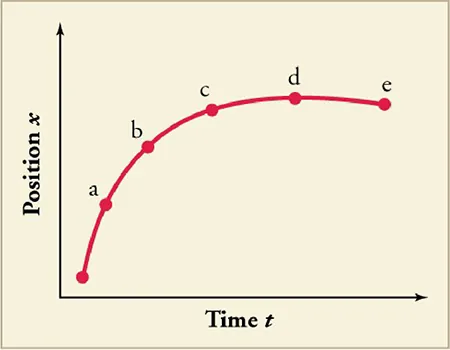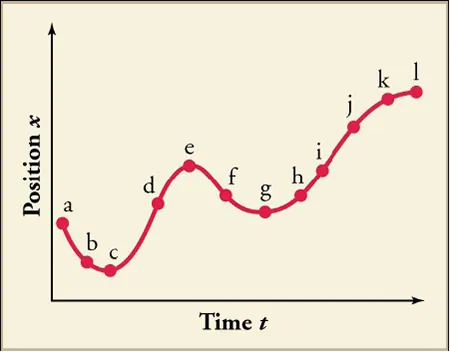Physics

# Critical Thinking Items

PhysicsCritical Thinking Items

### Critical Thinking Items

#### 2.1Relative Motion, Distance, and Displacement

9 .
Two boats are traveling at equal and opposite velocities when they pass each other. How would the captain of each boat describe the motion of the other boat?
1. Each captain would describe other boat’s motion the same because motion is independent of reference frame.
2. Each captain will describe other boat’s motion as moving in the backward direction because they are in their own reference frames.
3. Each captain would describe the boats as moving differently because motion is a relative term.
4. Each captain would describe the other boat’s motion perpendicularly because they will perceive them as moving opposite to each other.
10 .
Passenger A sits inside a moving train and throws a ball vertically upward. How would the motion of the ball be described by a fellow train passenger B and an observer C who is standing on the platform outside the train?
1. Passenger B sees that the ball has vertical, but no horizontal, motion. Observer C sees the ball has vertical as well as horizontal motion.
2. Passenger B sees the ball has vertical as well as horizontal motion. Observer C sees the ball has the vertical, but no horizontal, motion.
3. Passenger B sees the ball has horizontal but no vertical motion. Observer C sees the ball has vertical as well as horizontal motion.
4. Passenger B sees the ball has vertical as well as horizontal motion. Observer C sees the ball has horizontalbut no vertical motion.

#### 2.2Speed and Velocity

11 .
Is it possible to determine a car’s instantaneous velocity from just the speedometer reading?
1. No, it reflects speed but not the direction.
2. No, it reflects the average speed of the car.
3. Yes, it sometimes reflects instantaneous velocity of the car.
4. Yes, it always reflects the instantaneous velocity of the car.
12.

Terri, Aaron, and Jamal all walked along straight paths. Terri walked 3.95 km north in 48 min. Aaron walked 2.65 km west in 31 min. Jamal walked 6.50 km south in 81 min. Which of the following correctly ranks the three boys in order from lowest to highest average speed?

1. Jamal, Terri, Aaron
2. Jamal, Aaron, Terri
3. Terri, Jamal, Aaron
4. Aaron, Terri, Jamal
13 .
Rhianna and Logan start at the same point and walk in the same direction. If Rhianna’s average velocity is vavg,R and she walks for half of Logan’s time, what is Logan’s velocity vavg,L if he walks three times as far?
1. $v_{\text{avg,L}} = \frac{3}{2} v_{\text{avg,R}}$
2. $v_{\text{avg,L}} = \frac{2}{3} v_{\text{avg,R}}$
3. $v_{\text{avg,L}} = \sqrt{3 v_{\text{avg,R}}}$
4. $v_{\text{avg,L}} = 3 v_{\text{avg,R}}^{2}$

#### 2.3Position vs. Time Graphs

14.

Explain how you can use the graph of position vs. time to describe the change in velocity over time.Identify the time (ta, tb, tc, td, or te) at which at which the instantaneous velocity is greatest, the time at which it is zero, and the time at which it is negative.

#### 2.4Velocity vs. Time Graphs

15.

Identify the time, or times, at which the instantaneous velocity is greatest, and the time, or times, at which it is negative. A sketch of velocity vs. time derived from the figure will aid in arriving at the correct answers.1. The instantaneous velocity is greatest at f, and it is negative at d, h, I, j, and k.
2. The instantaneous velocity is greatest at e, and it is negative at a, b, and f.
3. The instantaneous velocity is greatest at f, and it is negative at d, h, I, j, and k
4. The instantaneous velocity is greatest at d, and it is negative at a, b, and f.
Order a print copy

As an Amazon Associate we earn from qualifying purchases.